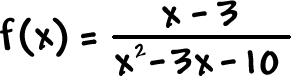X-INTERCEPTS Where the graph crosses the x-axis.

Hey, this is the same as finding the zeros!

 Setand solve

But, here's the cool thing:
For these rational guys, you just need to set the numerator =
0 and solve!

Well, when a fraction is 0, it's the same as saying the numerator is 0: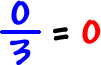So, set the numerator = 0 and solve.

Check it out: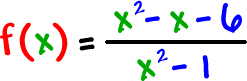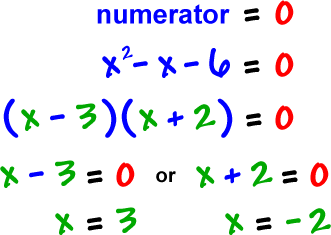So, the x-intercepts are the points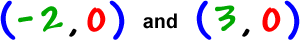Find the x-intercepts of